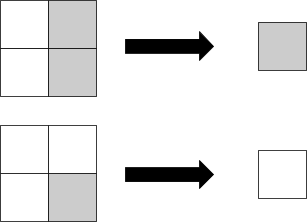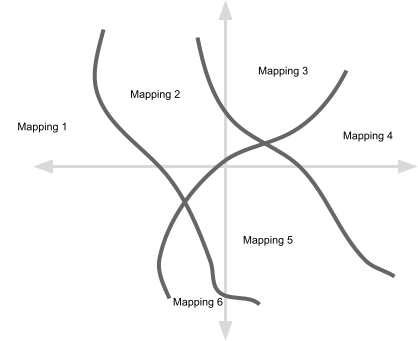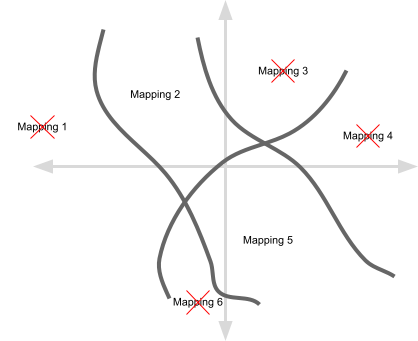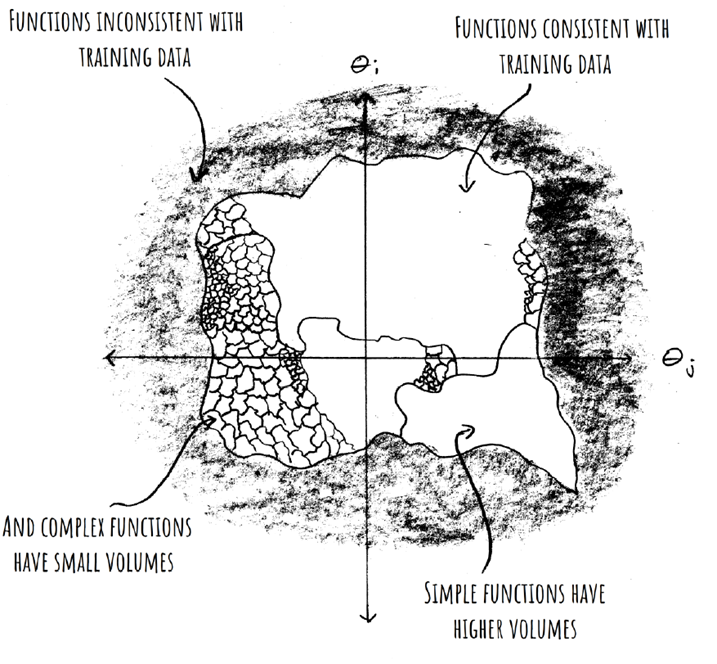# 32

Kolmogorov ComplexityAI
Frontpage

This is independent research. To make further posts like this possible, please consider supporting me.

Epistemic status: This is my understanding of multiple years of technical work by several researchers in just a few days of reading.

## Outline

• I attempt to summarize some of Chris Mingard’s recent work on why neural networks generalize so well.

• I examine one chunk of work that argues that mappings with low Kolmogorov complexity occupy large volumes in neural network parameter space.

• I examine a second chunk of work that argues that standard neural network training algorithms select mappings with probability proportional to their volume in parameter space.

## Introduction

During the 2000s, very few machine learning researchers expected neural networks to be an important part of the future of their field. Papers were rejected from major machine learning conferences with no reason given other than that neural networks were uninteresting to the conference. I was at a computer vision conference in 2011 at which there was a minor uproar after one researcher suggested that neural networks might replace the bespoke modelling work that many computer vision professors had built their careers around.

But neural networks have in fact turned out to be extremely important. Over the past 10 years we have worked out how to get neural networks to perform well at many tasks. And while we have developed a lot of practical know-how, we have relatively little understanding of why neural networks are so surprisingly effective. We don’t actually have many good theories about what’s going on when we train a neural network. Consider the following conundrum:

1. We know that large neural networks can approximate almost any function whatsoever.

2. We know that among all the functions that one might fit to a set of data points, some will generalize well and some will not generalize well.

3. We observe that neural networks trained with stochastic gradient descent often generalize well on practical tasks.

Since neural networks can approximate any function whatsoever, why is it that practical neural network training so often selects one that generalizes well? This is the question addressed by a recent series of papers by Chris Mingard.

The basic up-shot of Chris’ work, so far as I can tell, is the following:

• The optimization methods that we use to train neural networks are more likely to select mappings that occupy large volumes of neural network parameter space than functions that occupy small volumes of neural network parameter space.

• Most of the volume of neural network parameter space is occupied by simple mappings.

These are highly non-obvious results. There is no particular reason to expect neural networks to be set up in such a way that their parameter space is dominated by simple mappings. The parameter space of polynomial functions, for example, is certainly not dominated by simple mappings.

Chris’ work consists of a combination of empirical and theoretical results that suggest but do not decisively prove the above claims. In this post I will attempt to explain my understanding of these results.

## Simple mappings occupy larger volumes in parameter space

Chris’ work is all about volumes occupied by different functions in parameter space. To keep things simple, let’s consider a machine learning problem in which the inputs are tiny 2x2 images with each pixel set to 0 or 1, and the output is a single 0 or 1:Since there are 4 input pixels and each one can be either a 0 or a 1, there are 16 possible inputs. Each one of those inputs could be mapped to either a 0 or 1 as output, so there are 2^16 = 65,536 possible mappings from inputs to outputs. Any neural network with four input neurons and one output neuron is going to express one of these 65,536 possible mappings. We could draw out the whole space of possible neural network parameters and label each point in that space according to which of the 65,536 mappings it expresses:Each point in the above diagram represents a particular setting of the parameters in a neural network. I have drawn just two dimensions but there will be far more parameters than this. And I have drawn out volumes for 6 mappings but we would expect all 65,536 mappings to show up somewhere within the parameter space.

So given the picture above, we can now ask: do each of the 65,536 mappings occupy equal-sized volumes? Or do some occupy larger volumes than others? And if some mappings do occupy larger volumes than others then is there any pattern to which mappings occupy larger versus smaller volumes?

Chris’ work suggests that some mappings do in fact occupy larger volumes than others, and that it is the mappings with low Kolmogorov complexity that occupy larger volumes. What does it mean for a mapping to have a low Kolmogorov complexity? It means that there is a short computer program that implements the mapping. For example, the mapping that outputs 0 if there are an even number of black pixels in the input image and otherwise outputs 1 has a low Kolmogorov complexity because this mapping can be computed by XOR’ing all the input pixels together, whereas the mapping that outputs 0 for some randomly chosen arrangements of input pixels and otherwise outputs 1 has high Kolmogorov complexity because any computer program that computes this mapping will have to include a big lookup table within its source code. It is important to understand that when we talk about complexity we are talking about the length of a hypothetical computer program that would compute the same mapping that a given neural network computes. Also, (John reminds us)[https://www.lesswrong.com/posts/5p4ynEJQ8nXxp2sxC/parsing-chris-mingard-on-neural-networks?commentId=fzkGYmHsKdFx5dyzb] that the paper uses a proxy for simplicity that is actually pretty different from Kolmogorov complexity.

In order to demonstrate this, Chris worked with the well-known MNIST dataset, which contains images of handwritten digits of 28x28 pixels. This means that the number of possible images is 2^56, since in this dataset there are two possible pixel values, and the number of possible mappings is 10(256), since in this dataset there are 10 possible outputs. This is a very large number, which makes it infeasible to explore the entire space of mappings directly. Also, Kolmogorov complexity is uncomputable. So there was quite a bit of analytical and experimental work involved in this project. This work is summarized in the blog post "Deep Neural Networks are biased, at initialisation, towards simple functions", with references to the underlying technical papers. The conclusions are not definitive but they are highly suggestive, and they suggest that mappings with lower Kolmogorov complexity occupy relatively larger volumes in parameter space.

This sheds some light on the question of why trained neural networks generalize well. We expect that mappings with low Kolmogorov complexity will generalize better than mappings with high Kolmogorov complexity, due to Occam’s razor, and it seems that mappings with low Kolmogorov complexity occupy larger parameter space volumes than mappings with high Kolmogorov complexity.

## Mappings occupying larger parameter space volumes are more likely to be selected

The next question is: do the optimization algorithms we use to train neural networks care at all about the volume that a given mapping occupies in parameter space? If the optimization algorithms we use to train neural networks are more likely to select mappings that occupy large volumes in parameter space then we are one step closer to understanding why neural networks generalize, since we already have evidence that simpler mappings occupy larger volumes in parameter space, and we expect simpler mappings to generalize well. But they might not be more likely to select mappings that occupy large volumes in parameter space. Optimizations algorithms are designed to optimize, not to sample in an unbiased way.

A second blog post by Chris summarizes further empirical and theoretical work suggesting that yes, the optimization algorithms we use to train neural networks are in fact more likely to select mappings occupying larger volumes in parameter space. That blog post is called "Neural networks are fundamentally Bayesian", but it seems to me that viewing this behavior as Bayesian, while reasonable, is actually not the most direct way to understand what’s going on here.

What is really going on here is that within our original parameter space we eliminate all mappings except for the ones that perfectly classify every training image. We don’t normally train to 100% accuracy in machine learning but doing so in these experiments is a nice way to simplify things. So our parameter space now looks like this:The question is now: for the mappings that remain, is the standard neural network training algorithm (stochastic gradient descent) more likely to select mappings that occupy larger volumes in parameter space?

To investigate this, Chris compared the following methods for selecting a final set of neural network parameters:

1. Select neural network parameters at random until we find one that perfectly classifies every image in our training set, and output those parameters.

2. Train a neural network using the standard neural network training algorithm (stochastic gradient descent) and output the result.

We know that method 1 is more likely to select mappings that occupy larger volumes in parameter space because it is sampling at random from the entire parameter space, so a mapping that occupies twice the parameter space volume as some other mapping is twice as likely to be selected. So by comparing method 1 to method 2 we can find out whether practical neural network training algorithms have this same property.

But actually running method 1 is infeasible since it would take too long to find a set of neural network parameters that perfectly classify every image in the training set if sampling at random, so much of the work that Chris did was about finding a good approximation to method 1. To read about the specific methods that Chris used, see the blog post linked above and the technical papers linked from that post.

The basic picture that emerges is nicely illustrated in this graphic from the blog post linked above:## Scalability

Both of the claims discussed above are supported by a mixture of theoretical and empirical results. The empirical results are based on machine learning tasks that are relatively small-scale. This is understandable because the experiments involve re-training networks hundreds of thousands of times from scratch, which would be very expensive for the largest networks and problems being tackled today. However, it leaves open the question of whether these results will continue to hold as we run experiments with larger-scale networks and problems.

For further discussion of the likely reach of the results discussed here see this excellent post and its associated comments.

## Relevance to AI safety

If we want to align contemporary machine learning systems, we need to understand how and why those systems work. There is a great deal of work in machine learning that aims to find small "tips and tricks" for improving performance on this or that dataset. This kind of work does not typically shed much light on how or why our basic machine learning systems work, and so does not typically help move us towards a solution to the alignment problem. Chris’ work does shed light on how and why our basic machine learning systems work. It also provides an excellent example of how to perform the kind of empirical and theoretical work sheds light on how and why our basic machine learning systems work. I am excited to follow further developments in this direction.

1. the output neuron will be treated as a 1 if is positive or a 0 otherwise ↩︎

# 32

New Comment

Minor note: I think "mappings with low Kolmogorov complexity occupy larger volumes" is an extremely misleading way to explain what the evidence points to here. (Yes, I know Chris used that language in his blog post, but he really should not have.) The intuition of "simple mappings occupy larger volumes" is an accurate summary, but the relevant complexity measure is very much not Kolmogorov complexity. It is a measure which does not account for every computable way of compressing things, only some specific ways of compressing things.

In a sense, the results are more interesting in this light, since they tell us something about which specific ways of compressing things are relevant to our particular world. Since we cannot compute all the various ways of compressing things (i.e. Kolmogorov complexity and basically everything adjacent to it is uncomputable), this is a useful thing to know.

Thanks for this clarification John.

In a sense, the results are more interesting in this light, since they tell us something about which specific ways of compressing things are relevant to our particular world

But did Mingard et al show that there is some specific practical complexity measure that explains the size of the volumes occupied in parameter space better than alternative practical complexity measures? If so then think we would have uncovered an even more detailed understanding of which mappings occupy large volumes in parameter space, and since neural nets just generally work so well in the world, we could say that we know something about what kind of compression is relevant to our particular world. But if they just used some practical complexity measure as a rough proxy for Kolmogorov complexity then it leaves open the door for mappings occupying large volumes in parameter space to be simple according to many more ways of compressing things than were used in their particular complexity measure.

Yeah, I don't think there was anything particularly special about the complexity measure they used, and I wouldn't be surprised if some other measures did as-well-or-better at predicting which functions fill large chunks of parameter space.

For reasons elaborated upon in this post and its comments, I'm kinda skeptical of these results. Basically the claims made are

(A) That the parameter->function map is "biased towards simple functions". It's important to distinguish simple --> large volume and large volume --> simple. Simple --> large volume is the property that Solomonoff induction has and what makes it universal, but large volume-->simple is what is proven in these papers(plus some empirical evidence of unclear import)

(B) SGD being equivalent to random selection. The evidence is empirical performance of Gaussian processes being similar to neural nets on simple tasks. But this may break down on more difficult problems(link is about the NTK, not GP, but they tend to perform similarly, indeed NTK usually performs better than GP)

Overall I think it's likely we'll need to actually analyze SGD in a non-kernel limit to get a satisfactory understanding of "what's really going on" with neural nets.

Thanks for these pointers.

but large volume-->simple is what is proven in these papers(plus some empirical evidence of unclear import)

Is that the empirical evidence attempts to demonstrate simple --> large volume but is inconclusive, or is it that the empirical evidence does not even attempt to demonstrate simple --> large volume?

The evidence is empirical performance of Gaussian processes being similar to neural nets on simple tasks.

Well they do take many samples from what they call P_SGD and P_B and compare these as distributions, so it seems a little unfair to say that the evidence is that the performance is similar, since that would suggest that they were just comparing max performance by SGD to max performance by NNGP.

Re breaking down on more difficult problems: yes, I agree, we will have to wait and see and we shouldn't be too optimistic given the paper you point to in your own post.

[from your post on NTK/GP not learning features] it seems possible that they [neural networks] could be doing something much more interesting -- perhaps even implementing something like a simplicity prior over a large class of functions, which I'm pretty sure NTK/GP can't be

It sounds like you do think there is some chance that neural network generalization is due to an architectural bias towards simplicity. I would be very interested in your take on other (non-Mingard) research on this overall question if you have time to jot down some notes.

This comment I'm writing is mostly because this prompted me to attempt to see how feasible it would be to computationally enumerate the conditions for the weights of small networks like the 2 input 2 hidden layer 1 output in order to implement each of the possible functions. So, I looked at the second smallest case by hand, and enumerated conditions on the weights for a 2 input 1 output no hidden layer perceptron to implement each of the 2 input gates, and wanted to talk about it. This did not result in any insights, so if that doesn't sound interesting, maybe skip reading the rest of this comment. I am willing to delete this comment if anyone would prefer I do that.

Of the 16 2-input-1-output gates, 2 of them, xor and xnor, can't be done with the perceptrons with no hidden layer (as is well known), for 8 of them, the conditions on the 2 weights and the bias for the function to be implemented can be expressed as an intersection of 3 half spaces, and the remaining 6 can of course be expressed with an intersection of 4 (the maximum number that could be required, as for each specific input and output, the condition on the weights and bias in order to have that input give that output is specified by a half space, so specifying the half space for each input is always enough).

The ones that require 4 are: the constant 0 function, the constant 1 function, return the first input, return the second input, return the negation of the first input, and return the negation of the second input.

These seem, surprisingly, among the simplest possible behaviors. They are the ones which disregard at least one input. It seems a little surprising to me that these would be the ones that require an intersection of 4 half spaces.

I haven't computed the proportions of the space taken up by each region so maybe the ones that require 4 planes aren't particularly smaller. And I suppose with this few inputs, it may be hard to say that any of these functions are really substantially more simple than any of the rest of them. Or it may be that the tendency for simpler functions to occupy more space only shows up when we actually have hidden layers and/or have many more nodes.

Here is a table (x and y are the weights from a and b to the output, and z is the bias on the output):

outputs for the different inputs   when this function is computed
0000 (i.e. the constant 0)    z<0, x+y+z<0, x+z<0, y+z<0
0001 (i.e. the and gate)    x+y+z>0, x+z<0, y+z<0
0010 (i.e. a and not b)     z<0, x+y+z<0, x+z>0
0011 (i.e. if input a)     z<0, x+y+z>0, x+z>0, y+z<0
0100 (i.e. b and not a)     z<0, x+y+z<0, y+z>0
0101 (i.e. if input b)     z<0, x+y+z>0, x+z<0, y+z>0
0110 (i.e. xor)       impossible
0111 (i.e. or)       z<0, x+z>0, y+z>0
1000 (i.e. nor)       z>0, x+z<0, y+z<0
1001 (i.e. xnor)      impossible
1010 (i.e. not b)      z>0, x+y+z<0, x+z>0, y+z<0
1011 (i.e. b->a )      z>0, x+y+z>0, x+z<0
1100 (i.e. not a)      z>0, x+y+z<0, x+z<0, y+z>0
1101 (i.e. a->b )      z>0, x+y+z>0, y+z<0
1110 (i.e. nand )      x+y+z<0, x+z>0, y+z>0
1111 (i.e. constant 0)     z>0, x+z>0, y+z>0, x+y+z>0

Very very cool. Thank you for this drocta. What would it take to map out the sizes of the volumes corresponding to each of these mappings? Also, could you perhaps compute the exact Kolmogorov complexity of these mappings in some particular description language, since they are so small? It would be super interesting to me to assemble a table of volumes and Kolmogorov complexities for each of these small mappings. It may then be possible to write some code that does the same for 3-input and 4-input mappings.